Thread: Eric Dollard View Single Postt-rexSenior Member Join Date: Aug 2011 Location: In the bushes Posts: 422
Law of Electro-Magnetic Induction, Twelve. (2 of 4)

(6) Let the instantaneous value of Magnetism be given by the relation,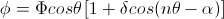Where,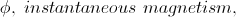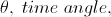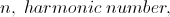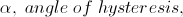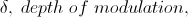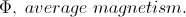Substituting the following relation for Magnetic Induction,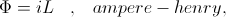Into the general expression segregates the two subjects of parameter variation, that of the Current, i, and that of the Inductance, L. The sine wave of current is given by,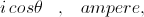And the pulsating wave of Inductance is given by,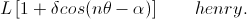Here in the Inductance the sine wave of variation is offset, and for a modulation depth of one (100%) the sine wave is a pulsating wave in variation with peaks at zero and twice the value of static Inductance. This is expressed as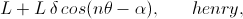Where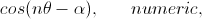Is the sine wave of Inductance variation. For a modulation depth of zero the cosine term vanishes and the constant term of static Inductance, L, remains,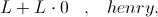Segregating variations the General Equations for Magnetic synchronous parameter variation becomes,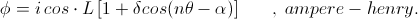(7) For the condition of Saturation and Hysteresis as exists in magnetic materials the process of saturation is the same for positive and negative half cycles, it is symmetrical. The reduction of Inductance due to saturation in the positive half is the same reduction of Inductance due to the saturation in the negative half. Hence the reduction, or modulation, of Inductance is at Twice the frequency of the A.C. cycle of Magnetism, that is, the Inductance pulsates at Double Frequency. In this situation it is,

n = 2 , numeric,

Where, n, is the harmonic number.
__________________
SUPPORT ERIC DOLLARD'S WORK AT EPD LABORATORIES, INC.

Purchase Eric Dollard's Books & Videos - 70% of the sale goes to Eric and EPD Laboratories: Eric Dollard Books & Videos
Donate by Paypal: Donate to EPD Laboratories